Courses

# Previous year questions (2014-19) - Waves NEET Notes | EduRev

## NEET : Previous year questions (2014-19) - Waves NEET Notes | EduRev

The document Previous year questions (2014-19) - Waves NEET Notes | EduRev is a part of the NEET Course Physics 28 Years Past year papers for NEET/AIPMT Class 11.
All you need of NEET at this link: NEET

Q 1. A tuning fork is used to produce resonance in a glass tube. The length of the air column in this tube can be adjusted by a variable piston. At room temperature of 27°C two successive resonances are produced at 20 cm and 73 cm column length. If the frequency of the tuning fork is 320 Hz, the velocity of sound in air at 27°C is:-    
A: 330 m/s
B: 339 m/s
C: 350 m/s
D: 300 m/s
Ans: B
Solution
: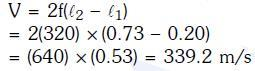Q 2. The fundamental frequency in an open organ pipe is equal to the third harmonic of a closed organ pipe. If the length of the closed organ pipe is 20cm, the length of the open organ pipe is:-    
A: 13.2 cm
B: 8 cm
C: 12.5 cm
D: 16 cm
Ans:
A
Solution: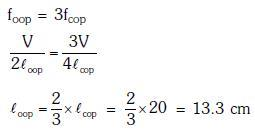Q 3. The two nearest harmonics of a tube closed at one end and open at other end are 220 Hz and 260 Hz. What is the fundamental frequency of the system?    
A: 20 Hz
B: 30 Hz
C: 40 Hz
D: 10 Hz
Ans:
A
Solution:
Two successive frequencies of closed pipe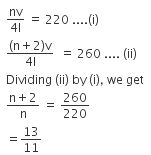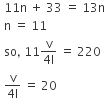Q 4. Two cars moving in opposite directions approach each other with speed of 22 m/s and 16.5 m/s respectively. The driver of the first car blows a horn having a a frequency 400 Hz. The frequency heard by the driver of the second car is [velocity of sound 340 m/s]:-    
A: 361 Hz
B: 411 Hz
C: 448 Hz
D: 350 Hz
Ans:
C
Solution: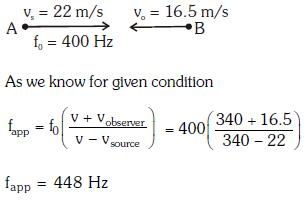Q 5. A siren emitting a sound of frequency 800 Hz moves away from an observer towards a cliff at a speed of 15 ms-1. Then, the frequency of sound that the observer hears in the echo reflected from the cliff is : (Take velocity of sound in air = 330 ms-1)    
A: 885Hz
B: 765 Hz
C: 800 Hz
D: 838 Hz
Ans:
D
Solution: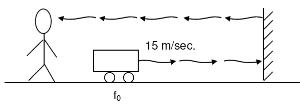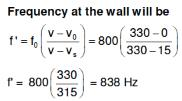Since the observer and the wall are stationary so frequency of echo observed by the observer will also be 838 Hz.

Q 6. An air column, closed at one end and open at the other, resonantes with a tuning fork when the smallest length of the column is 50 cm. The next larger length of the column resonating with the same tuning fork is:    
A: 200 cm
B: 66.7 cm
C: 100 cm
D: 150 cm
Ans:
D
Solution:
The smallest length of the air column is associated with the fundamental mode of vibration of the air column.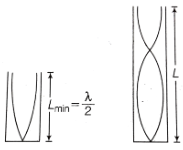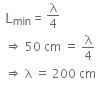The next higher length of the air column is,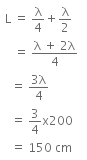Q 7. A uniform rope of length L and mass m1 hangs vertically from a rigid support. A block of mass m2 is attached to the free end of the rope. A transverse pulse of wavelength λ1 is produced at the lower end of the rope. The wavelength of the pulse when it reaches the top of the rope is λ2. The ratio λ12 is :    
A: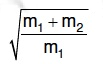B: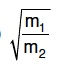C: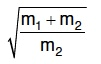D: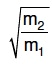Ans:
C
Solution: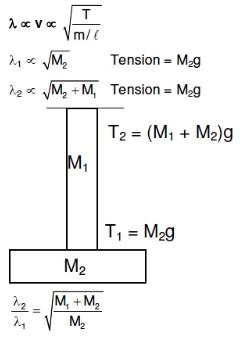Q 8. The fundamental frequency of a closed organ pipe of length 20 cm is equal to the second overtone of an organ pipe open at both the ends. The length of organ pipe open at both the ends is:    
A: 140 cm
B: 80 cm
C: 100 cm
D: 120 cm
Ans:
D
Solution:
For closed organ pipe fundamental frequency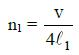For open organ pipe fundamental frequency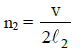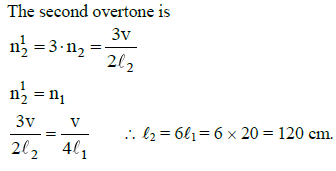Q 9. If n1, n2, and n3 are the fundamental frequencies of three segments into which a string is divided, then the original fundamental frequency n of the string is given by:    
A: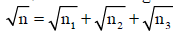B: n = n1 + n2 + n3
C: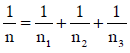D

: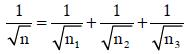Ans: C
Solution: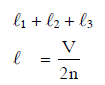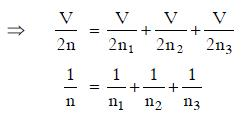Q 10. The number of possible natural oscillations of air column in a pipe closed at one end of length 85 cm whose frequencies lie below 1250 Hz are : (velocity of sound = 340 ms−1)    
A: 7
B: 6
C: 4
D: 5
Ans: B
Solution: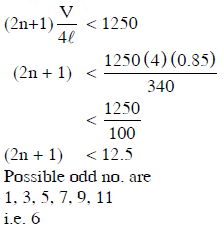Q 11. A speeding motorcyclist sees traffic jam ahead of him. He slows down to 36 km/hour. He finds that traffic has eased and a car moving ahead of him at 18 km/hour is honking at a frequency of 1392 Hz. If the speed of sound is 343 m/s, the frequency of the honk as heard by him will be:    
A: 1412 Hz
B: 1454 Hz
C: 1332 Hz
D: 1372 Hz
Ans:
A
Solu
tion: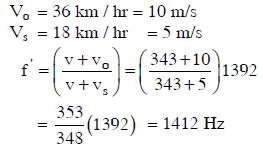Offer running on EduRev: Apply code STAYHOME200 to get INR 200 off on our premium plan EduRev Infinity!

14 docs|25 tests

,

,

,

,

,

,

,

,

,

,

,

,

,

,

,

,

,

,

,

,

,

;